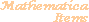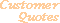Home Older Mathematica Items

Scientific Arts

# Single Electron Atom Wave Functions

Page 1 | Page 2 | Page 3

## Visualization

There is endless variety in the ways that one can visualize these wave functions. Here we write a few functions that automate the process of plotting these functions.

The first function, PlotYlm, provides a spherical polar plot of the angular part of the wave function. In addition to the options for the ParametricPlot3D function, PlotYlm can take on three other options. The option Orbitals determines whether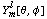or the linear combination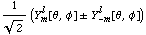is plotted. In the latter case the option Parity specifies the choice in the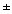sign. The option ShowQuantumNumbers determines whether or not the graphic includes information on the angular momentum quantum numbers.  The coloring of the graphic was chosen rather arbitrarily (in the color directive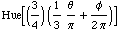): it is fun to experiment with other possibilities.

``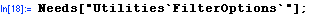``
``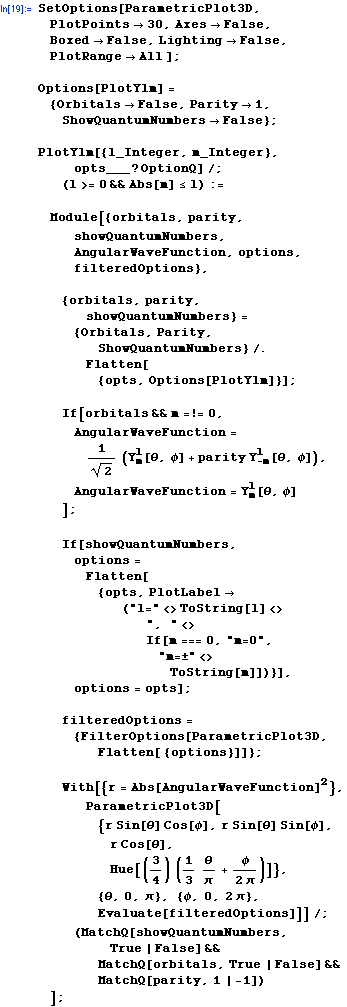``

The function MakeYlmGraphicsArray plots an array of angular wave functions. An example is shown after the function definition.

``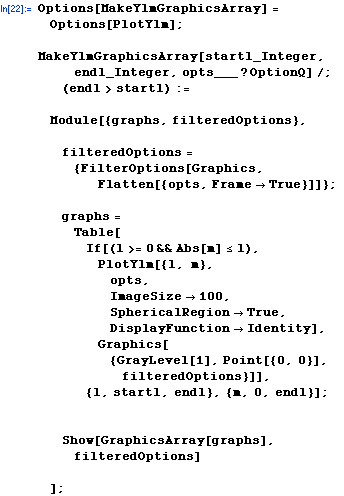``

Here is an example of MakeYlmGraphicsArray.

``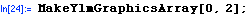``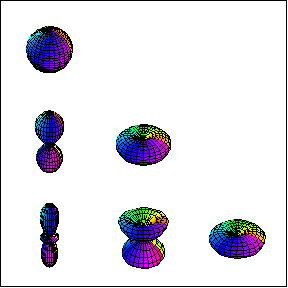Here is a 3-dimensional plot of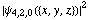in the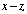plane.

``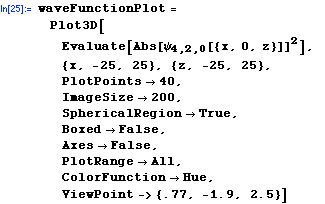``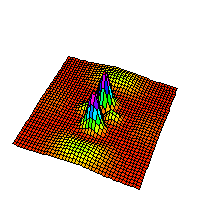`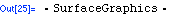`

A SurfaceGraphics object can be turned into an equivalent ContourGraphics object simply by wrapping it in the ContourGraphics function.

``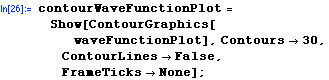``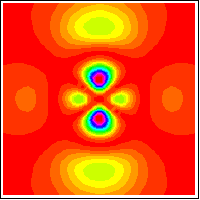Here we create plots of the absolute squares of the radial and angular wave function. The display of these plots is suppressed by using the option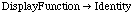.

``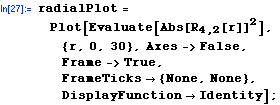``

Now the angular plot.

````

All three plots can be shown together by using Rectangle and GraphicsArray:

``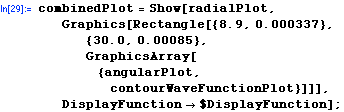``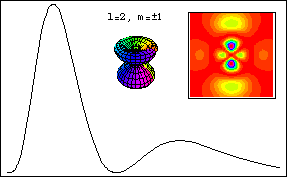One could go on to create a function that automatically creates a graphic of this type.

### ExtendGraphics package use

Here is an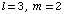odd parity orbital:

``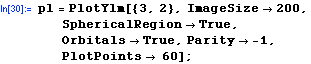``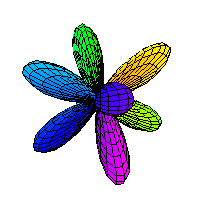We can alter the visual display of this with some of the functions contained in the ExtendGraphics` set of packages that were written by Tom Wickham-Jones and are described in his book "Mathematica Graphics". The ExtendGraphics` packages are available free of charge from MathSource.

``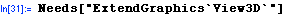``

These functions from ExtendGraphics` alter the lighting properties.

``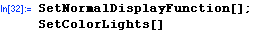``

Now redisplay the graphic. To see the effects of the lighting modifications we need to set the Lighting option to True (it was set to False within the definition of PlotYlm).

``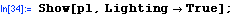``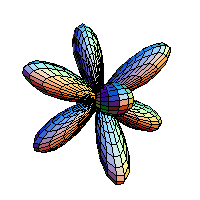The following uses pattern matching and a replacement rule to take this graphic and remove the surface mesh.

````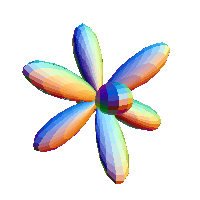As we stated earlier, the possibilities for visualization are endless. The various three dimensional graphics above can also be animated with Mathematica (by plotting the graphics from various ViewPoints, or by using the standard add-on package Graphics`Animation`, or by a variety of other means). The results can be exported as animated GIFs, and so on.

## <<Previous

 For further information on our services send email to info@scientificarts.com . Contents of this web site Copyright © 1999-2011, Scientific Arts, LLC.
 s